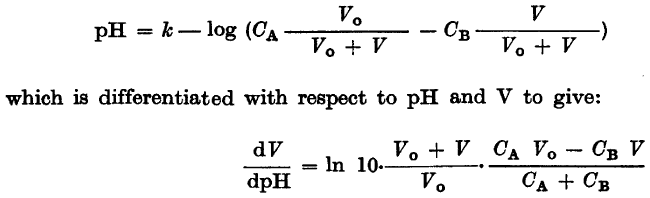# Dx/dy vs dy/dx

Mentor
I am reading an old paper and I have came to the passage shown in the picture:k, CA, CB and V0 are all just some constants.

I think I know what they did - they differentiated the first equation calculating dpH/dV and then took reciprocals of both sides of the equation. Is it a valid approach?

I am not able to get the same result solving the first equation for V and calculating dV/dpH then.

Bear in mind I am mathemathically challenged and English is my second language, I can be missing something obvious.

Last edited:

D H
Staff Emeritus
Yes, this is a valid approach. Let $y=f(x)$. Obviously, $dy/dx = df/dx$. Differentiating $y=f(x)$ with respect to y yields

$$1 = \frac{df}{dx}\,\frac{dx}{dy}$$

Solving for $dx/dy$,

$$\frac{dx}{dy} = \frac 1 {df/dx} = \frac 1 {dy/dx}$$

Mentor
OK, thank you.

So going the other way (that is solving for V and differentiating), I should get the same result?

epenguin
Homework Helper
Gold Member
OK, thank you.

So going the other way (that is solving for V and differentiating), I should get the same result?

On the way there I think you slightly simplify and easier see the calculation if you combine terms inside the bracket of first eq. into a single fraction and then express that as

pH = k - log(CAV0 - CBV) + log(V0 + V)Mentor
I have to solve for V, having two logs doesn't make it easier.

I tried both manually and with TI 89, but I can't get the same result as the one printed. Will try later again.

epenguin
Homework Helper
Gold Member
Borek, I'll put this up here instead of PM as hopefully someone can check it in case of mistakes er typos.For the differentiation

$$pH = k - log(\frac{C_AV_0 - C_BV}{V_0 + V})$$

$$= k - log(C_AV_0 - C_BV) +log(V_0 + V)$$

$$= k + \ln 10 [-\ln(C_AV_0 - C_BV) + \ln(V_0 + V)]$$

Then differentiating

$$\frac{dpH}{dV} = [\frac{- C_B}{C_AV_0 - C_BV} + \frac{1}{V_0 + V}].\ln 10$$

From this you can already see that the point where the LHS is infinite (the pH against V graph is vertical, or V against pH horizontal) is where
$$V = \frac{V_0C_A}{C_B}$$.

The RHS fractions can be combined giving

$$[\frac{\ln10.(C_A - C_B)V_0}{(C_AV_0 - C_BV)(V_0 + V)}]$$

If you wanted to invert this into V = a function of $$\frac{dV}{dpH}$$, rearrange to

$$(C_AV_0 - C_BV)(V_0 + V) = (C_A - C_B)V_0.\ln 10.\frac{dV}{dpH}$$

call the RHS R, say, and then express V by solving the unlovely quadratic in V:

$$-V^2C_B + V V_0(C_A - C_B) + (C_A V_0^2 - R) = 0$$

You ought to get the same thing by inverting and differentiating but I think it is more tedious.

You said you want to invert the first equation anyway. I question whether you do- if it is for plotting it suffices to switch around axes when you plot rather than invert algebraically.

For inverting algebraically work with H rather than pH; and instead of k (which is probably a p something) I define k = - log M, (M = 10-k)

Then the first equation becomes

$$H = M + \frac{C_AV_0 - C_BV}{V_0 + V}$$

which eventually transforms to

$$V = [\frac{C_A - C_B}{C_B + H - M} + 1]V_0$$

or in terms of pH and k

$$V = [\frac{C_A - C_B}{C_B + 10^{-H} - 10^{-k}} + 1]V_0$$

Last edited:
Mentor
From this you can already see that the point where the LHS is infinite (the pH against V graph is vertical, or V against pH horizontal) is where
$$V = \frac{V_0C_A}{C_B}$$.

Hardly surprising - that's the equivalence pointYou said you want to invert the first equation anyway.

Whatever I was doing was just to make sure I understand what is going on. And today I had a time to try again - everything is OK.

call the RHS R, say, and then express V by solving the unlovely quadratic in V:

$$-V^2C_B + V V_0(C_A - C_B) + (C_A V_0^2 - R) = 0$$

Something is wrong, no need for quadratic here:

$$V = \frac {-(10^k - C_A 10^{pH}) V_0} {10^k + C_B 10^{pH}}$$

For inverting algebraically work with H rather than pH

This is from the Gran method original paper, BTW.

k (which is probably a p something)

No, that's log(activity coefficient).

Thank's for the help. Yesterday I was not sure about this reciprocal thing - it seemed logical, but I have learnt not to trust my instincts when it comes to anything more complicated than multiplication table.

Last edited:
epenguin
Homework Helper
Gold Member
I will work through it again with more calm and clearer head (also had computer trouble today).We want to show the students how we make mistakes and are very 'umble.Redbelly98
Staff Emeritus
Homework Helper
Borek, I'll put this up here instead of PM as hopefully someone can check it in case of mistakes er typos.For the differentiation

$$pH = k - log(\frac{C_AV_0 - C_BV}{V_0 + V})$$

$$= k - log(C_AV_0 - C_BV) +log(V_0 + V)$$

$$= k + \ln 10 [-\ln(C_AV_0 - C_BV) + \ln(V_0 + V)]$$

Then differentiating

$$\frac{dpH}{dV} = [\frac{- C_B}{C_AV_0 - C_BV} + \frac{1}{V_0 + V}].\ln 10$$
Should that be +CB in the numerator (two minus-signs cancel each other)?